Question

# A spherical water drop 1.6 um in diameter is suspended in calm air due to a...

A spherical water drop 1.6 um in diameter is suspended in calm air due to a downward-directed atmospheric electric field of magnitude E = 1000 N/C.

(a) What is the magnitude of the gravitational force on the drop? (b) How many excess electrons does it have?

(a) Number  _______  Units _______

(b) Number  _______  Units _______

SOLUTION :

a.

Volume of water drop = 4/3 pi r^3 = 4/3 pi (d/2)^3 = pi d^3 / 6

= pi * (1.6 * 10^(-6)) ^3 / 6

=  1.6^3 * pi / 6 *10^(-18)

= 2.14466 * 10^(-18)  m^3

Density of water = 1000 kg/m^3

So, mass of water = 1000 * 2.14466 * 10^(-18) = 2.14466 * 10^(-15) kg

So, gravitational force on the drop

= m*g

= 2.14466 *10^(-15) * 9.8  (N)

b.

In equilibrium, force of electric field , Fe = Gravitational force, Fg

=> Number of electrons * per electron force * Electric field magnitude = 2.1017668 * 10^(-14)

=> Number of electrons * 1.6 * 10^(-19) * 1000  = 2.1017668 * 10^(-14)

=> Number of electrons = 2.1017668 * 10^(-14) / (1.6 * 10^(-16)

=> Number of electrons = 131 (ANSWER)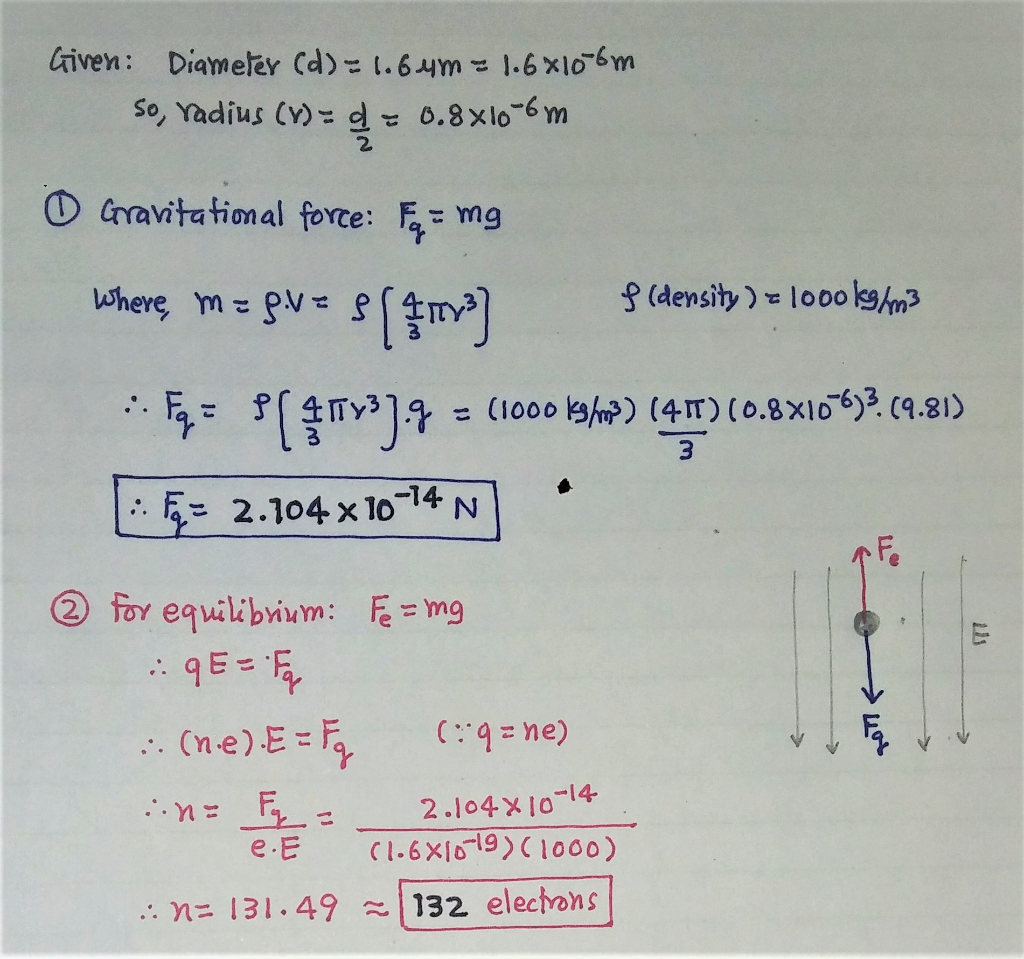first part is wrong

source: wiley

#### Earn Coins

Coins can be redeemed for fabulous gifts.

Similar Homework Help Questions
• ### A spherical water drop, 1.60 μm in diameter, is suspended in calm air owing to a...

A spherical water drop, 1.60 μm in diameter, is suspended in calm air owing to a downward-directed atmospheric electric field E = 492 N/C. What is the weight of the drop? How many excess electrons does the drop have?

• ### A small drop of water is suspended motionless in air by a uniform electric field that...

A small drop of water is suspended motionless in air by a uniform electric field that is directed upward and has a magnitude of 8200 N/C. The mass of the water drop is 3.81x 10^-9 kg. (a) Is the excess charge on the water drop positive or negative? Why? (b) How many excess electrons or protons reside on the drop?

• ### A small drop of water is suspended motionless in air by a uniform electric field that...

A small drop of water is suspended motionless in air by a uniform electric field that is directed upward and has a magnitude of 8110 N/C. The mass of the water drop is 2.72 × 10-9 kg. How many excess electrons or protons reside on the drop?

• ### A small drop of water is suspended motionless in air by a uniform electric field that...

A small drop of water is suspended motionless in air by a uniform electric field that is directed upward and has a magnitude of 12000 N/C. The mass of the water drop is 3.23 × 10-9 kg. How many excess electrons or protons reside on the drop?

• ### A small drop of water is suspended motionless in air by a unilform electric field that...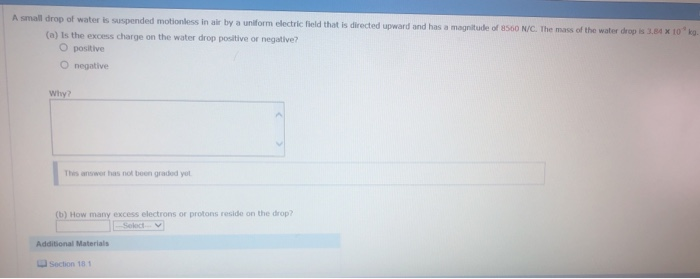A small drop of water is suspended motionless in air by a unilform electric field that is directed upward and has a a magnitude of 8560 N/C. The mass of the water drop is 3.64 x 10ko. (a) Is the excess charge on the water drop positive or negative? O positive O negative This answer has not been graded yo (b) How many excess electrons or protons reside on the drop? Additional Materials Lu Section 18

• ### 66. A small drop of water is suspended motionless in air by a uniform electric field...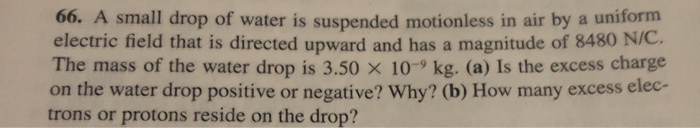66. A small drop of water is suspended motionless in air by a uniform electric field that is directed upward and has a magnitude of 8480 N/C. The mass of the water drop is 3.50 × 10-9 kg. (a) is the excess charge on the water drop positive or negative? Why? (b) How many excess elec- trons or protons reside on the drop?

• ### In the famous Millikan oil-drop experiment, tiny spherical droplets of oil are sprayed into a uniform...

In the famous Millikan oil-drop experiment, tiny spherical droplets of oil are sprayed into a uniform vertical electric field. The drops get a very small charge (just a few electrons) due to friction with the atomizer as they are sprayed. The field is adjusted until the drop (which is viewed through a small telescope) is just balanced against gravity and therefore remains stationary. Using the measured value of the electric field, we can calculate the charge on the drop and...

• ### In the famous Millikan oil-drop experiment, tiny spherical droplets of oil are sprayed into a uniform...

In the famous Millikan oil-drop experiment, tiny spherical droplets of oil are sprayed into a uniform vertical electric field. The drops get a very small charge (just a few electrons) due to friction with the atomizer as they are sprayed. The field is adjusted until the drop (which is viewed through a small telescope) is just balanced against gravity and therefore remains stationary. Using the measured value of the electric field, we can calculate the charge on the drop and...

• ### In Millikan's experiment, an oil drop of radius 1.89 um and density 0.874 g/cm3 is suspended...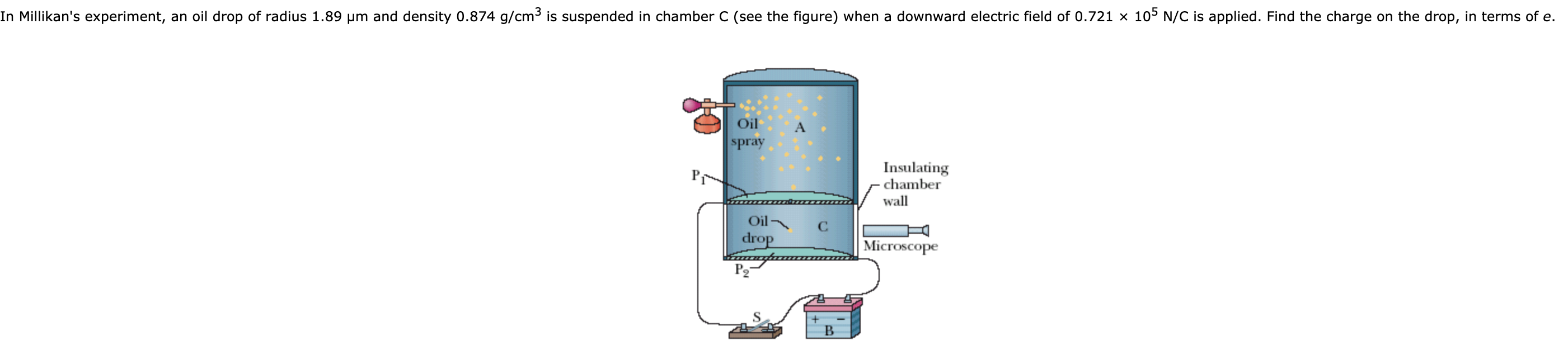In Millikan's experiment, an oil drop of radius 1.89 um and density 0.874 g/cm3 is suspended in chamber C (see the figure) when a downward electric field of 0.721 x 105 N/C is applied. Find the charge on the drop, in terms of e. Oil spray P1 Insulating chamber wall Oil drop С Microscope P2 B

• ### The classic Millikan oil drop experiment was the first to obtain an accurate measurement of the...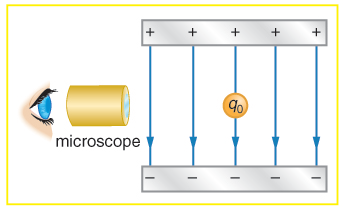The classic Millikan oil drop experiment was the first to obtain an accurate measurement of the charge on an electron. In it, oil drops were suspended against the gravitational force by a vertical electric field. Consider an oil drop with a weight of 1.6 x 10-14N, if the drop has a single excess electron, find the magnitude (in N/C) of the electric field needed to balance its weight. Your should round your answer to an integer, indicate only the number,...